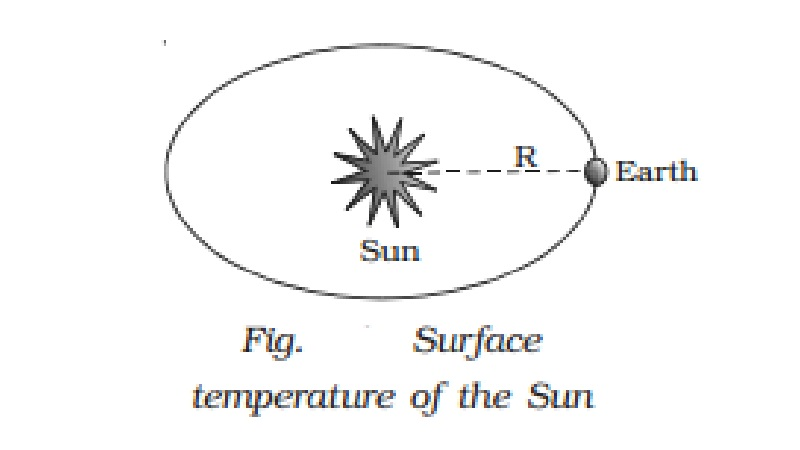Home | | Physics | | Physics | Surface temperature of the Sun

# Surface temperature of the SunThe solar constant is the amount of radiant energy received per second per unit area by a perfect black body on the Earth with its surface perpendicular to the direction of radiation from the sun in the absence of atmosphere.

Solar constant

The solar constant is the amount of radiant energy received per second per unit area by a perfect black body on the Earth with its surface perpendicular to the direction of radiation from the sun in the absence of atmosphere. It is denoted by S and its value is 1.388 ? 103 W m-2. Surface temperature of the Sun can be calculated from solar constant.

Surface temperature of the Sun

The Sun is a perfect black body of radius r and surface temperature T. According to Stefan?s law, the energy radiated by the Sun per second per unit area is equal to σT4.

Where σ is Stefan?s Constant.

Hence, the total energy radiated per second by the Sun will be given by

E = surface area of the Sun ? σT4

Let us imagine a sphere with Sun at the centre and the distance between the Sun and Earth R as radius (Fig.).  The heat energy from the Sun will necessarily pass through this surface of the sphere.

If S is the solar constant, the amount of heat energy that falls on this sphere per unit time is E = 4πR2S          ????.(2)

By definition, equations (1) & (2) are equal.

4πr2σT4. = 4πR2S

T4 = R2S/r2σ

T = (R/r)1/2(S/ σ)1/4

Knowing the values of R, r, S and σ the surface temperature of the Sun can be calculated.

Study Material, Lecturing Notes, Assignment, Reference, Wiki description explanation, brief detail

Related Topics